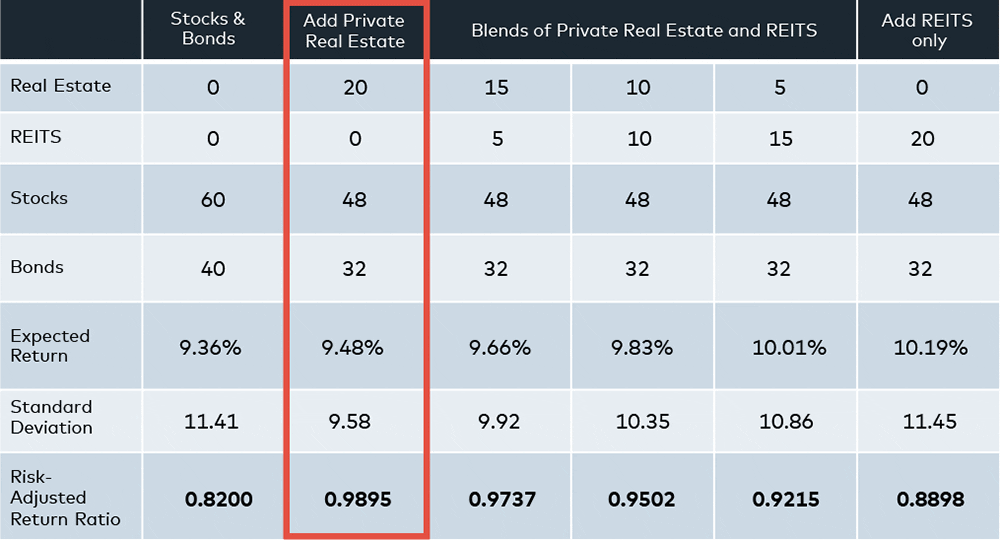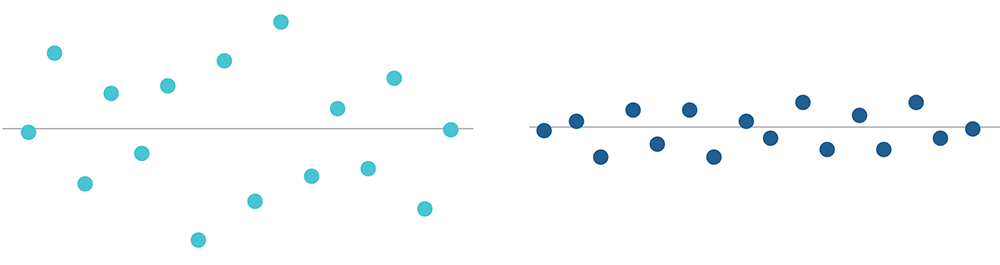# Improve Your Portfolio’s Returns, Lower Your Volatility with Private Real EstateThe chart above makes a strong, data-supported argument for adding private real estate to a portfolio of stocks and bonds.

Of all of the combinations listed, stocks and bonds plus private real estate provides the best return for the level of risk involved — a 0.9895 risk-adjusted return ratio — and the lowest level of volatility or standard deviation, 9.58.

Low volatility, high expected returns and low correlation are the three most important variables when considering adding any asset to a portfolio. And according to TIAA CREF, private real estate, as measured by the NCREIF real estate index, has little to no correlation to stocks and bonds.

“Putting the S&P 500 and the Dow Jones Industrial Average together is not diversifying,” said Michael Episcope, Origin’s cofounder. “There is no benefit to the overall portfolio because these indexes are nearly perfectly correlated to one another. The addition of private real estate enhances any traditional portfolio of stocks and bonds because of its low correlation to these asset classes and high expected returns.”

Financiers understand these two charts. The rest of us, however, require some guidance.

Get real estate investing articles twice a month.

First some definitions.

Standard deviation.

This is a measure of volatility. OK. What does that mean?

Glance at the two scatter plots below. The scatter on the left is spread out, while the scatter on the right is bunched up. Yet both have the same center line. The scatter on the left, thus, has a higher standard deviation, or dispersion around the average, than the one on the right.It is far more likely that any point on the left scatter is farther away from the average than any point on the right one. Therefore, overall, the left scatter is much more volatile or subject to fluctuation.

Many investors prefer less volatility and want investment products that exhibit low standard deviations.

Expected return.

The expected return is akin to that middle line. It is the return one most expects to receive based on historical data.

Its calculation is heavily influenced by the probability of a set of outcomes. Let’s say, based on historical data from the last five years, a stock has a 50 percent chance of increasing in value by 5 percent and a 50 percent chance of losing 2 percent.

The expected return is (50% * 5%) + (50% * -2%) = 0.015 = 1.5%

But a 1.5 percent return isn’t guaranteed; it’s only the most likely outcome. So how should you analyze it? According to Investopedia, use the expected return to determine whether, on average, an investment offers a positive or negative return.

This is a more refined version of the expected return as it now takes into account how much risk is required to get that 1.5 percent expected return explained above.

There are five important measures of risk, but the one used most in real estate investing is the Sharpe ratio. This is the toughest measure on this chart to explain.

We’ve got to start with the risk-free rate, which financiers consider a zero-risk investment, and is equal to the expected return on a U.S. Treasury bill or bond. This investment, as a baseline, has a Sharpe ratio of zero.

“The Sharpe ratio is the average return in excess of the risk-free rate per unit of volatility or total risk,” Investopedia explains. “Generally, the greater the value of the Sharpe ratio, the more attractive the risk-adjusted return.”

So thinking about this, can you guess one number that needs to be in this calculation? Well, volatility is what, again? Standard deviation. And what part of this investment return do we care about? We want to take the risk-free rate out, as that’s a given return, and isolate the part of the return that’s associated with the risk.

So the equation is mean of the portfolio return minus the risk-free rate divided by the standard deviation of the portfolio return. This helps investors figure out what adding or subtracting a new investment to a portfolio does to its return, given the amount of risk that has been added, and that’s exactly what the final row of the original chart depicts.

Now, let’s walk through the rest of that chart. The first four lines show different mixes of the four asset classes analyzed:

• Column 1) stocks and bonds;
• Column 2) stocks and bonds plus private real estate;
• Column 3-5) stocks and bonds plus private real estate and publicly-traded real estate investment firms, known as REITS; and
• Column 6) stocks and bonds plus REITS.

In summary, honing in on Column 2, stocks and bonds plus private real estate delivers the lowest volatility and the best returns for the risk involved. It is also what we recommend to all of our clients.

## Posted ByOrigin Investments is a top-ranked real estate firm committed to educating investors on private equity commercial real estate.